# Box-Cox transformation

(Redirected from Box–Cox transformation)

Transformations of data designated to achieve a specified purpose, e.g., stability of variance, additivity of effects and symmetry of the density. If one is successful in finding a suitable transformation, the ordinary method for analysis will be available. Among the many parametric transformations, the family in [a1] is commonly utilized.

Letbe a random variable on the positive half-line. Then the Box–Cox transformation ofwith power parameteris defined by: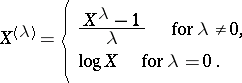The formula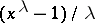is chosen so that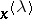is continuous astends to zero and monotone increasing with respect tofor any.

The power parameteris estimated by a graphical technique or by the maximum-likelihood method. Unfortunately, a closed form for the estimator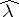can be rarely found. Hence, the plot of the maximum likelihood againstis helpful. The value ofobtained in this way is treated as if it were a true value, and then one fits the model to the transformed data. Such an approach may be easily carried out, and an asymptotic theory associated with other parameters is useful. See [a1] and [a3].

This treatment has, however, some difficulties becausehas a variability and depends on the given data itself. It is known that estimation ofby maximum likelihood and related likelihood-ratio tests can be heavily influenced by outliers (cf. also Outlier). Further, in certain situations, the usual limiting theory based on knowingdoes not hold in the unknown case. Therefore, several robust estimation procedures have been proposed (see Robust statistics; and [a5] and references therein).

In the literature, Box–Cox transformations are applied to basic distributions, e.g., the cubic root transformation of chi-squared variates is used for acceleration to normality (cf. also Normal distribution), and the square-root transformation stabilizes variances of Poisson distributions (cf. also Poisson distribution). These results are unified by appealing to features of the following family of distributions.

Consider a collection of densities of the formsatisfyingwith. This family is called an exponential dispersion model with power variance function (EDM-PVF) of index. The existence of such a model was shown in [a2] unless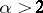or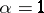. It is a flexible family, including the normal, Poisson, gamma-, inverse Gaussian, etc., distributions.

It is known that both of the normalizing and the variance-stabilizing transformations of the exponential dispersion model with power variance function are given by Box–Cox transformations, see [a4]. If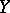follows the exponential dispersion model with power variance function and with index, the normalizing and variance-stabilizing transformations are given by, respectively, where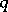(the power for normalization) and(the power for variance-stabilization) are summarized in the Table below (recall that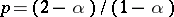). The similar characteristics of familiar distributions are also tabulated there. For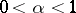, it has been proved in [a4] that the density ofhas a uniformly convergent Gram–Charlier expansion (cf. also Gram–Charlier series). This implies that the normalizing transformation which is obtained by reducing the third-order cumulant reduces all higher-order cumulants as a result (cf. also Cumulant).'
<tbody> </tbody>
 Distribution indexNormal 2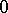Poisson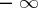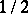Gamma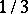Inverse GaussianEDM-PVF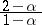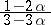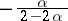Box–Cox transformations are also applied to link functions in generalized linear models. The transformations mainly aim to get the linearity of effects of covariates. See [a3] for further detail. Generalized Box–Cox transformations for random variables and link functions can be found in [a5].